## Everyday Docker tips

Docker has become one of my favorite tools to help with challenges of different build and run environments. When I want to create even a small example project, I usually start by creating first the Dockerfile for the build environment. When I have had to distribute more complex work for the client, images/containers have made the process almost painless.

I wanted to write down some of the things that have made my life much easier while using Docker.

## Basic recap

This is post is not intended as a full tutorial / getting started guide, but let’s recap quickly. Basic Dockerfile usually looks something like this:

# base image on top of which we build our own
FROM ubuntu:20.04

# Fetch package index and install packages
RUN apt-get update && apt-get install -y nano

# In which directory next commands will be applied.
# Also last WORKDIR will be CWD when the container is run
WORKDIR /data

# Copy data from the build directory to the image
COPY . /data

# Entrypoint is the default executable to be run when
# using the image
ENTRYPOINT ["ls", "-la"]


I recommend looking through the Docker documentation for the Dockerfile for a lot more options.

To build the image we usually run:

docker build -t name-of-the-image .


And finally we run the image:

# --rm = remove container when it is stopped
docker run --rm name-of-the-image


# List running and stopped containers
docker ps -a

# List disk space usage of Docker
docker system df -v

# Remove non running images/cache. Does not touch volumes (add --volumes to prune volumes too)
# Prompts for confirmation
docker system prune

# Monitor what is happening in the "docker system" by printing messages of started/exiting containers, networks etc
docker system events


## Mounting volumes with -v

One of the first things I wanted to do with docker was to modify my host machines file system. This can be easily achieved with mounting volumes / binding. Easy mistake to make is to assume that if you have directory data in your current directory, and you want to mount it to /data, that you could do this with

# Current PWD directory structure
# . .. data build
# Following will not mount data directory, but will create a
# named volume data:
docker run --rm -v data:/data name-of-the-image

# Clean up
docker volume ls
docker volume rm data


Correct way to bind directories is to always use full paths. When using scripts to run the container, this gets bit more tricky as user can have the script in any directory. Fortunately Unix tools help with this:

docker run --rm -v readlink -f data:/data name-of-the-image
# Or when running git bash in Windows
docker run --rm -v pwd -w/data:/data name-of-the-image


readlink -f relative-path will return the full path for the relative-path. In Windows when using git bash, the paths returned by readlink are mangled and it would be better to use PowerShell, or if only subdirectories need to be mounted, pwd -W will work.

## More robust way to apt-get update && apt-get install

Using just apt-get update && apt-get install will leave files in the final image and cause problems with caching and increase the image size. It is recommended to clean up after installing packages. Another good option to try out is adding --no-install-recommends to minimize number of additionally installed packages.

FROM ubuntu:20.04
RUN apt-get update && apt-get install -y --no-install-recommends \
build-essential && \
rm -rf /var/lib/apt/lists/*


## BuildKit

BuildKit is something that has been integrated into newish versions of Docker to help with the build process. Most notable feature, at least for me, has been how you can handle secrets with it. To use the BuildKit features, the docker build needs to be run with

DOCKER_BUILDKIT=1 docker build .


Or alternatively you can enable it in /etc/docker/daemon.json

#syntax = docker/dockerfile:1.2
FROM .....


On some earlier versions of Docker you might need to use line:

# syntax = docker/dockerfile:1.0-experimental
FROM .....


### Secrets

Sure way to shoot yourself in the foot is to copy any credentials into the image while building it. For example following will leak your ssh keys:

COPY .ssh /home/root/
RUN git clone git@github.com:some/internal_repo.git && \
<build> && \
rm -rf /home/root/.ssh


Correct way to use secrets while building the image is to use build in secrets sharing. This complicates the build command a bit, but things are easily fixed with small bash script:

#!/bin/bash
if [[ $_ ==$0 ]]
then
echo "Script must be sourced"
exit 1
fi

# To use ssh credentials (--mount=type=ssh), we need
# ssh-agent. Many desktop environments already have the
# service running in the background, but for more bare
# bones desktop envs might need to start the service
# separately.


## Multi-stage builds

One of the best ways to clean up the the image and save space is multi-stage builds. In multi-stage build data from previously build image is used to construct the final image.

# First stage containing common components
FROM ubuntu:20.04 as base
RUN apt-get update && apt-get install -y \
some dependencies && \
rm -rf /var/lib/apt/lists/*

# Second stage that builds the binaries
FROM base as build
RUN apt-get update && apt-get install -y \
build-essential cmake git && \
rm -rf /var/lib/apt/lists/*
WORKDIR /src
RUN git clone git@github.com:some/repo.git && \
cmake -S repo -B build && \
cmake --build build --parallel 12

# Third stage that is exported to final image
FROM base as final
COPY --from=build /src/build/bin /build/bin
EXECUTABLE ["/build/bin/executable"]


To make sure that the final image contains only data that was visible in the last layer, we can use --squash when building the image. This will produce image with only single layer.

docker build --squash -t name-of-the-image


Unfortunately even though the image size is small, it is often slower to download than multilayer image, as Docker will download multiple layers in parallel.

In a one case when trying to minimize image download time for an image which contained a 15GB layer produced by single RUN command, I created a python script which sliced the huge directory structure into multiple smaller directories and then used a multi stage build to copy and reconstruct the directory structure. This way the 15GB download was sped up significantly.

## No, I don’t want to choose the keyboard layout

Sometimes when installing packages the build process stops and prompts keyboard layout. To avoid this DEBIAN_FRONTEND env should be set:

FROM ubuntu:20.04
ARG DEBIAN_FRONTEND=noninteractive
RUN apt-get update && apt-get install -y --no-install-recommends \
i3 && \
rm -rf /var/lib/apt/lists/*


## Running graphical apps inside of the container

Sometimes it would be nice to run graphical apps inside the container. Fortunatley this is quite easy with x11docker.

curl -fsSL https://raw.githubusercontent.com/mviereck/x11docker/master/x11docker | sudo bash -s -- --update
x11docker x11docker/xfce xfce4-terminal


On Windows GWSL seems to be very easy to use.

GUI apps should also be possible on OSX, but for me xquartz has not worked really well even without Docker, so I have not tried this one out.

### GUI apps without x11docker on Linux

It’s possible to run GUI apps in Linux without x11docker, but it’s much more brittle:

docker run -it --rm --net=host -e DISPLAY=$DISPLAY --volume="$HOME/.Xauthority:/root/.Xauthority":ro x11docker/xfce xfce4-terminal


## Packaging complex application with Docker and Python script

When packaging an application with Docker, it would feel nice to just send the pull and run commands to the customer. But when the application requires mounting/binding volumes, opening ports, running GUI, it soon becomes nightmare to document and explain the run command for all the use cases.

I have started to write small Python scripts for running the container. My bash skills are bit limiting and every time I have written a bash script for running the container, I have ended up converting it to a Python script.

Here is quick example

#!/usr/bin/env python3

import argparse
import os

def get_image_name(args):
return 'name-of-the-image'

def get_volumes(args):
path = os.path.abspath(os.path.realpath(os.path.expanduser(args.data_path)))
if not os.path.exists(path):
raise RuntimeError('Path {} does not exists'.format(path))
cmd = '-v {path}:/data:ro'.format(path=path)
return cmd

def get_command(args):
return 'ls -la'

def run_docker(args):
cmd = 'docker run {volumes} {image_name} {command}'.format(
volumes=get_volumes(args),
image_name=get_image_name(args),
command=get_command(args))
os.system(cmd)

def main():
parser = argparse.ArgumentParser()
args = parser.parse_args()
run_docker(args)

if __name__ == '__main__':
main()


## Inspecting images and linting

Sometimes you want to get bit more information how the image has been build and what kind of data it contains. Fortunately there is some tools to do this.

Dive is command line tool that processes the image and can display what changed in each of the previous layers. As a Docker tool, it is of course available as a Docker image:

docker run --rm -it \
-v /var/run/docker.sock:/var/run/docker.sock \
wagoodman/dive:latest name-of-the-image-to-analyze


docker run --rm -i hadolint/hadolint < Dockerfile


This Hacker News post listed few other interesting looking tools, but I have had no change to really test them out.

## DIY Promaster/Peugeot Boxer/Citroen Relay/Jumper Roof Rack for Fiat Ducato 2018 model

I saw a post on Reddit asking how to build a roof rack on a Fiat Ducato type of a vehicle, so here is how we build ours on a Fiat Ducato L3H2 2018.

When converting our van, we did not want to spend much on the roof rack, and we did not value our time either, so we designed and build our own. The hardest part was making of the roof rack mounting brackets. They could be bought for about 20-30€ a piece, but you can also build them yourself from U-shaped aluminum profile.

Making one mounting bracket took me approximately 10-15 minutes after few hours of experimentation.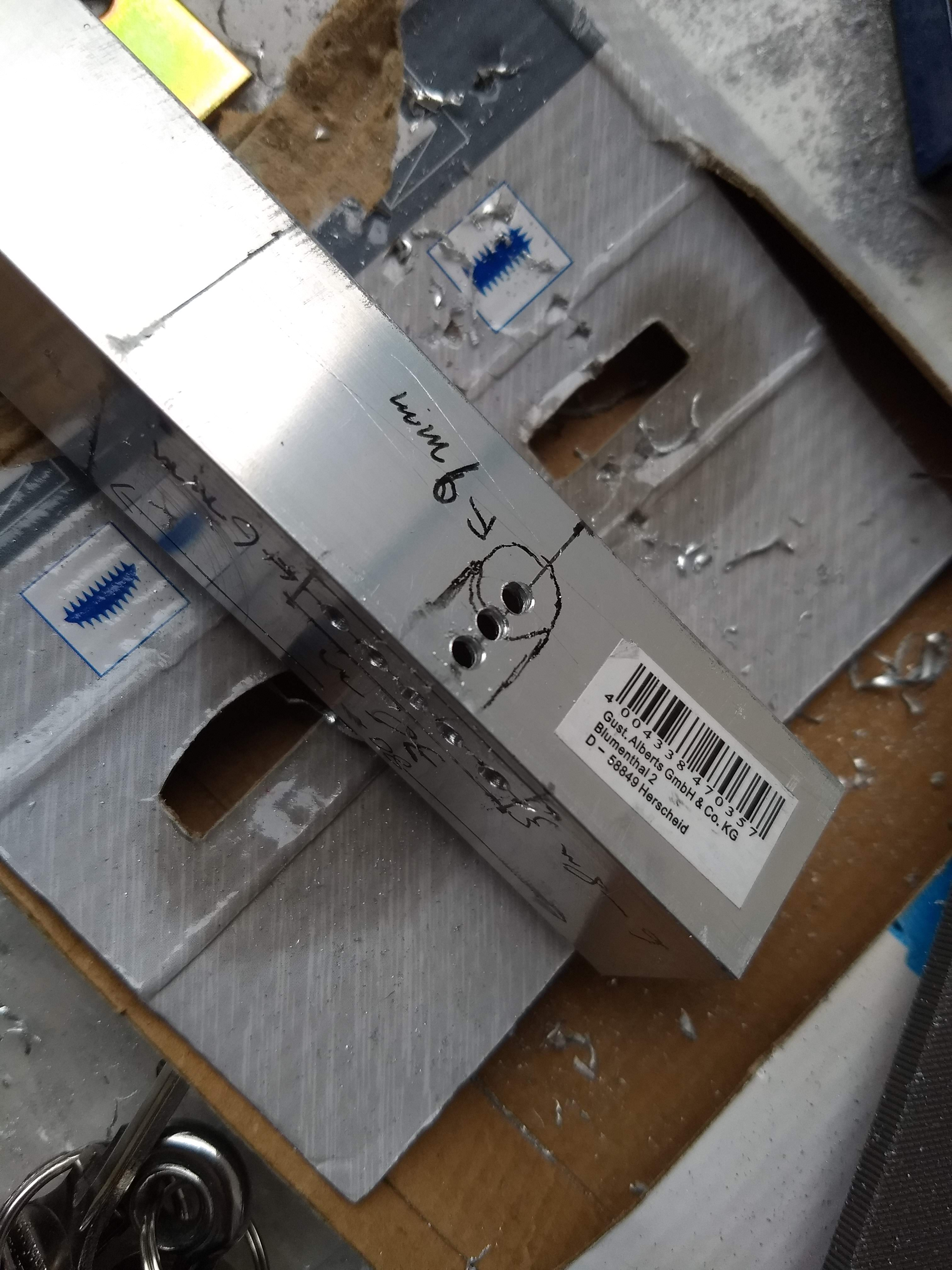It is not necessary to drill these smaller holes. I think I used 7.5mm for all the holes and then cleaned the rest with a very rough file. Much quicker.

Take approximately 80mm piece of ~2.5cm sturdy U-shaped aluminum profile and drill 7.5mm-9mm holes into strategic locations. Make the profile a bit lower with a jigsaw, if needed. This piece should be then inserted into slightly larger sized U-profile (2.5-3.0cm, you can bend the sides of the larger profile to make the fit looser). Then trill two holes through both of the profiles and insert two low profile bolts through the holes. Do not make the holes too big, as then the bolt will turn loosely and you can not tighten the bracket. Note that if inner profile is too high, you can not tighten the bracket on the roof as the inner part will hits the surrounding piece.

We added rubber sheet under the bracket to make sure that the roof is not ramaged.

The roof rack itself is made from 40x40mm profile from https://easy-systemprofile.de. There are many other alternative sources to use in Europe, but for us they seemed like a good compromise. For easier installation and cheaper postage we decided to cut the long profiles into two shorter sections and connect them with connectors. The profile lengths are 2x1900mm, 2x1600mm and 5x1500mm. 1500mm profiles could be approximately 10mm longer, as in our case the profiles are few millimeters too short and there is a small gab between the connections.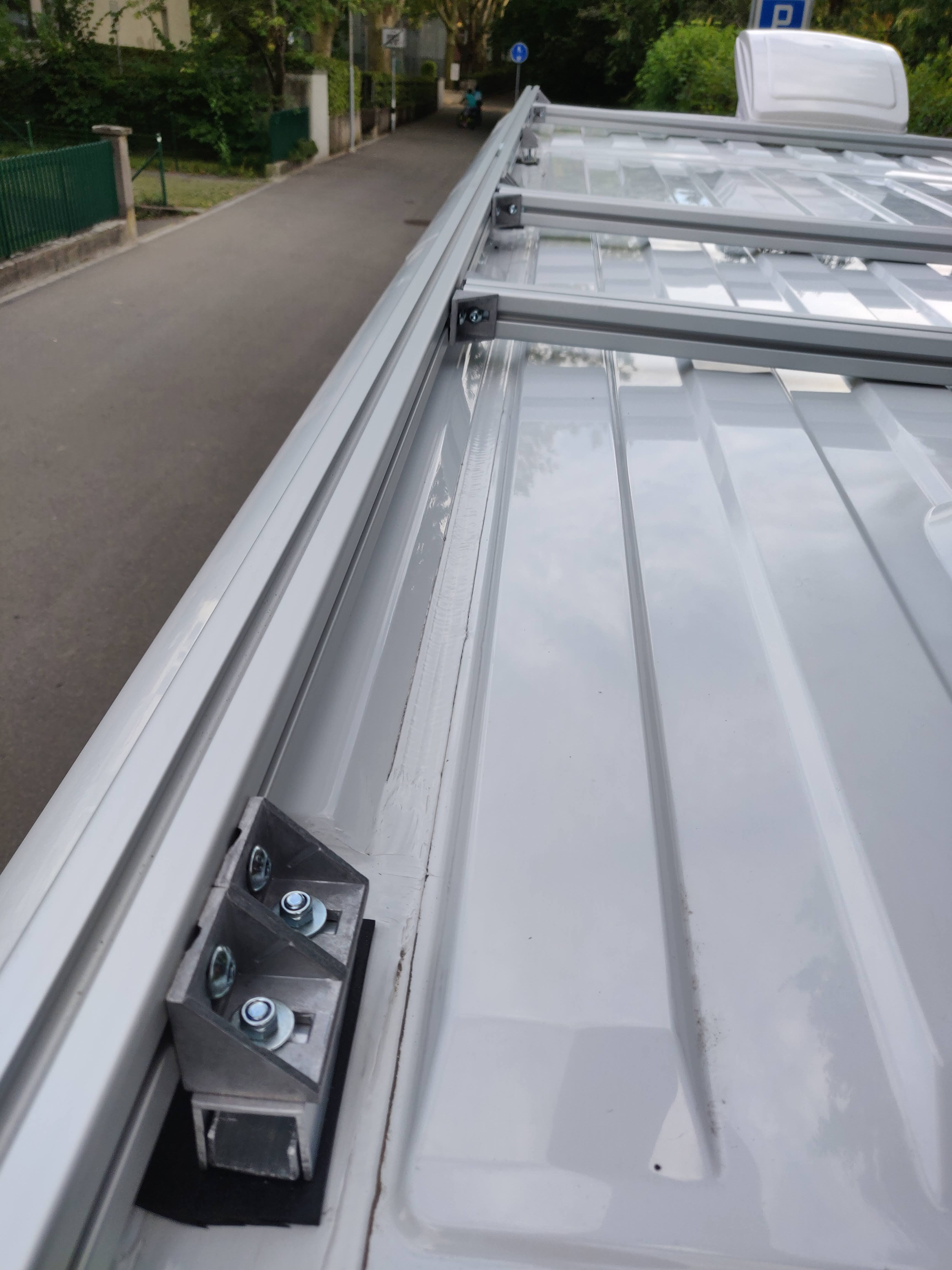The roof rack is bit too low (it can be lifted bit higher than in this image). Adding 1cm more height would make installing the solar panels easiers.

The end results is pretty sturdy and low profile and cost bit les than 300€ with all postages, nuts and bolts.

## Zurich water fountain map for running and biking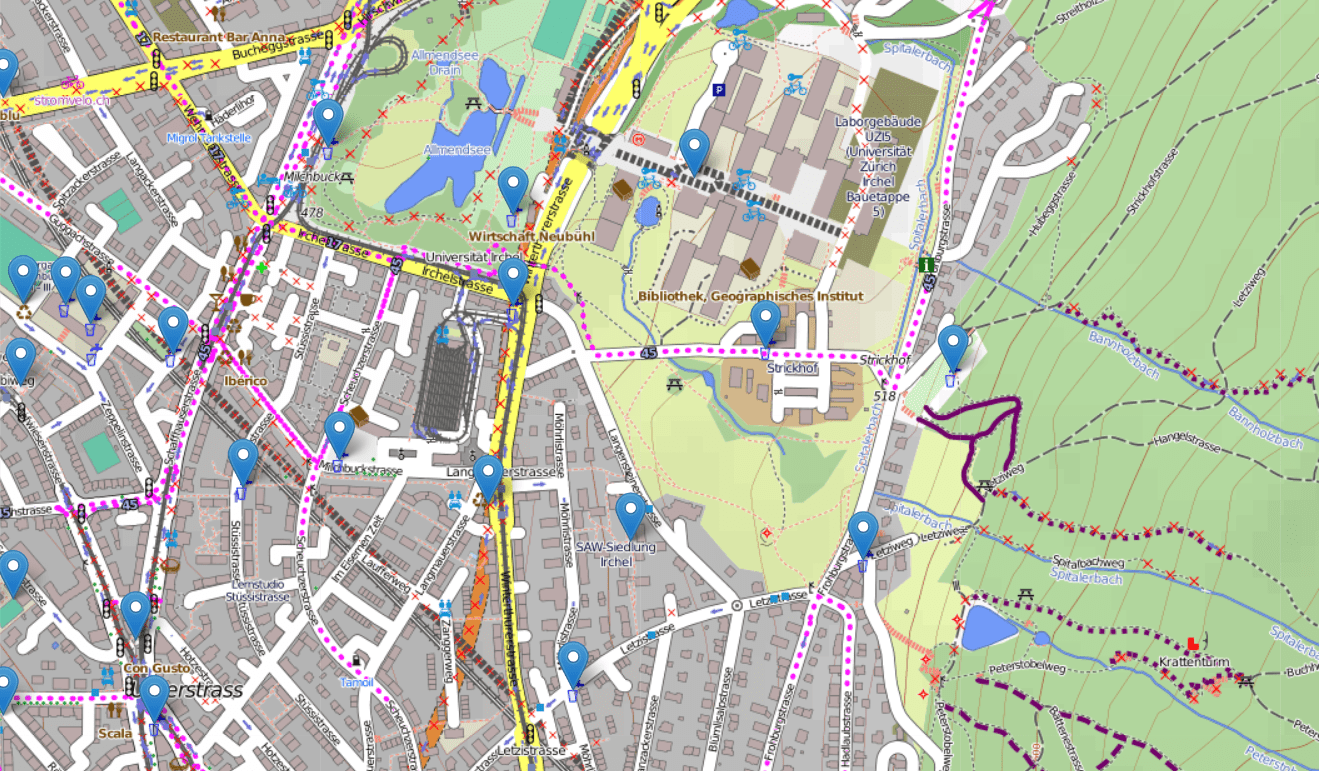As we moved to Zurich recently, I have been exploring the city by running. Many of the days have been pretty warm and we unfortunately misplaced the running belt while packing our stuff for the move. Fortunately Zurich has around 1200 public water fountains to drink from, and even better there is a dataset containing all the locations of the fountains (https://data.stadt-zuerich.ch/dataset/geo_brunnen). Matthew Moy has build a map (https://skyplan.ch/maps/zurich-water/) on top of the dataset which is pretty good, but the graphics are not designed for outdoor activities and centering to current location seems to only work once per page load.

As the data is open, I decided to build simple and pretty light weight map page which contains up to date (2020) locations of the water fountains: https://buq2.github.io/zurich-water-fountains-map/

The map graphics are more suited for outdoor activities and the page also draws the users route which hopefully helps when trying to orient yourself while trying to find nearest fountain. I hope some one else also finds the map useful.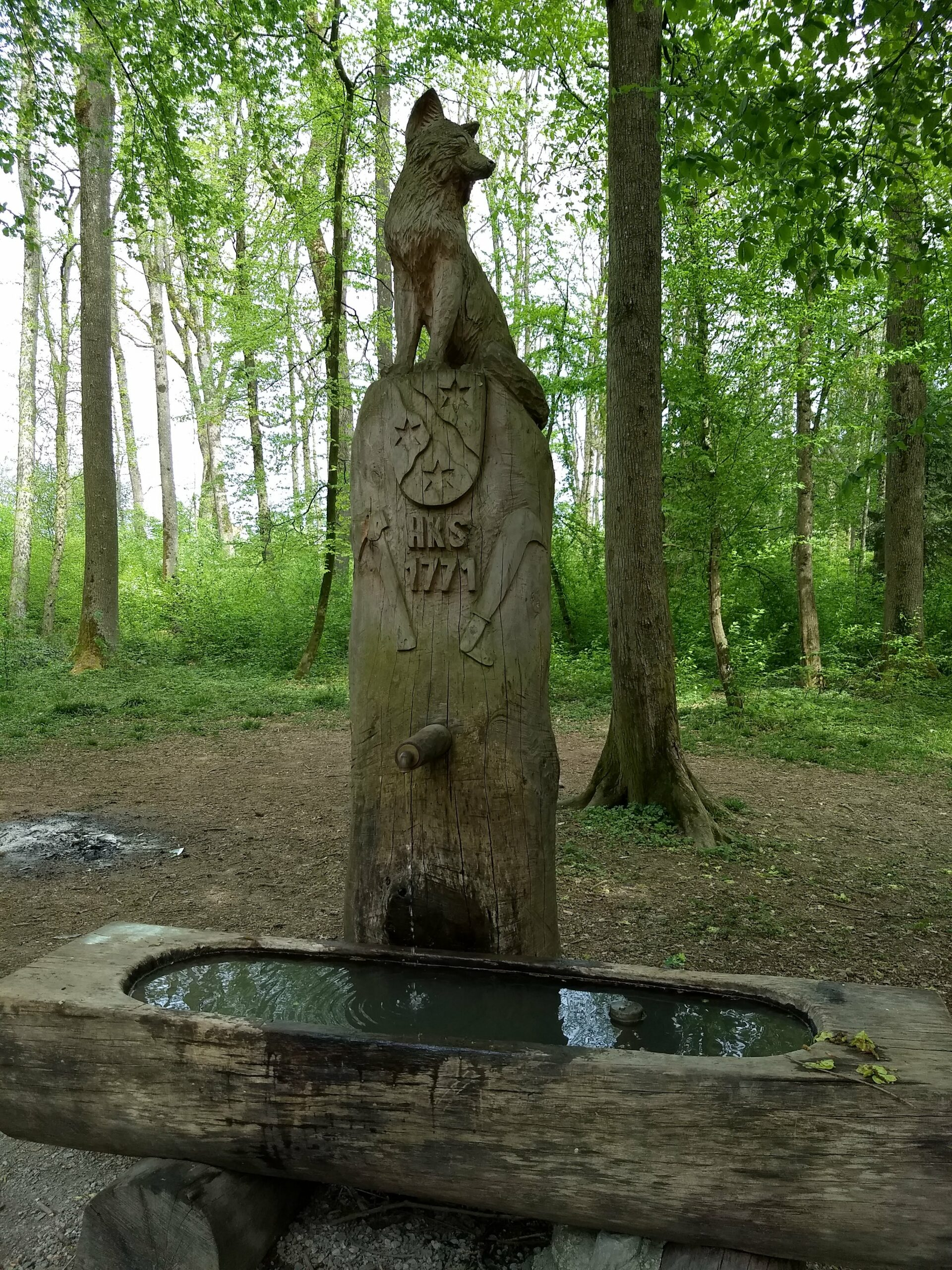## Learning to sew: Designing and creating a wingsuit – Ramblings

Few years ago I saw video (1) of a home build miniature parachute made by my friend Tuukka. The video inspired me quite a bit as before the video I didn’t feel like you could actually build any real “hardware” for skydiving without going to rigging courses and practicing a lot. I felt that designing and building parachute would be too much for my skills, but maybe I could still make something from fabrics.

In 2017 Tuukka jumped his home build and designed parachute (2).

At the end of 2016 skydiving season I borrowed a sewing machine, purchased cheap fabric and spend one evening sewing my very first tracking pants without any sewing patterns. The pants were huge failure (air intakes tore and the pants functioned as a air brakes), but hey, at least I created something.

During the winter I designed first tracking pants which I tested on the first jump of 2017. Unfortunately the design was not very good and the crotch seam and fabric was torn after two jumps while squatting on the ground.

Next there was a design for tracking jacket, but the design for the shoulders was so bad that I didn’t jump any jumps with the thing.

Finally third tracking pants+jacket design was relative good and I was able to have glide ratio around 1.1.

Natural progress towards diy wingsuit required diy one-piece tracking suit so I build one in late 2017. The suit was little bit hard to fly and I’m still not sure of performance of the suit. But at least it looked quite nice.

In December 2017 I started designing the diy wingsuit. I looked at beginner/advanced beginner level wingsuits from different manufacturers and draw a outline of the wingsuit in Inkscape. I used my friends Colugo 2 wingsuit to get idea of the details. Design was done once again in Clo3d/Clo. It took approximately 10-15 hours to design the suit. I ordered the pattern from a printing company which specializes in construction design drawings. This way I didn’t have to tape more than a hundred A4s together to get the full pattern.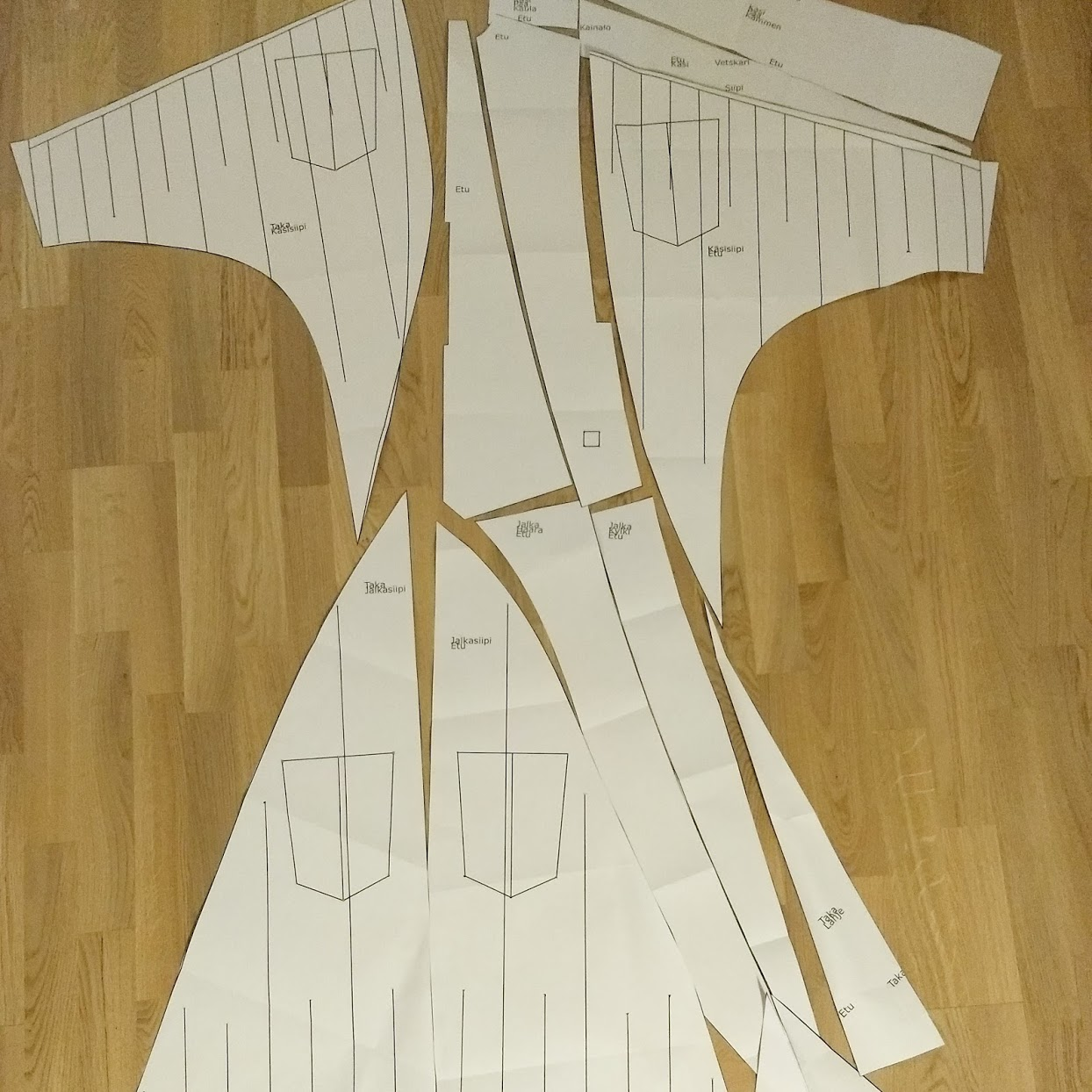Cutting the patterns took only about one hour. Cutting and sewing the first arm wing took about 9 hours, with the second one I made few stupid mistakes and had to unpick the half finished wing back to its basic components.Quite soon I realized that the leg wing had a design flaw as it did not extend over the buttocks as all commercial wingsuits. Unfortunately at this point modifications were too hard to make for my skills, but I decided to complete the suit.

After about total of 60-120 hours of work the suit was finished. The arm wings still were little bit rough as the sewing machine started to malfunction at the very last hours.

Finally in 2018-04-22 I did first jump with the wingsuit (also my very first wingsuit jump). The suit was very stable and the jump went well. In 2018 I had about 6 jumps with the wingsuit, every one of them quite stable (except when trying backflying). I can achieve around 1.8 glide ratio (wind compensated) with the suit, but I think the GL could be much better with practice.

Unfortunately when moving away from Finland, I had to leave the suit to storage as space in the van was very limited.

Hopefully I return to the suit at some point, maybe I will get few jumps with ‘real’ suits in between.

## Skydiving helmet chin mount update

I have got few questions about the chin mount for G3 and how has it been working. I have not used the mount for more than a year as the original mount was little bit too long and had poor contact with the G3 which caused wobbling. I felt that the base of the mount would have needed at least one or two updates to get it working well enough for daily use.

Unfortunately I didn’t have time to do the updates and people who have requested the original model files have not sent any updates back 🙂 I also started doing little bit more serious photography and I had to change the helmet to this monstrosity: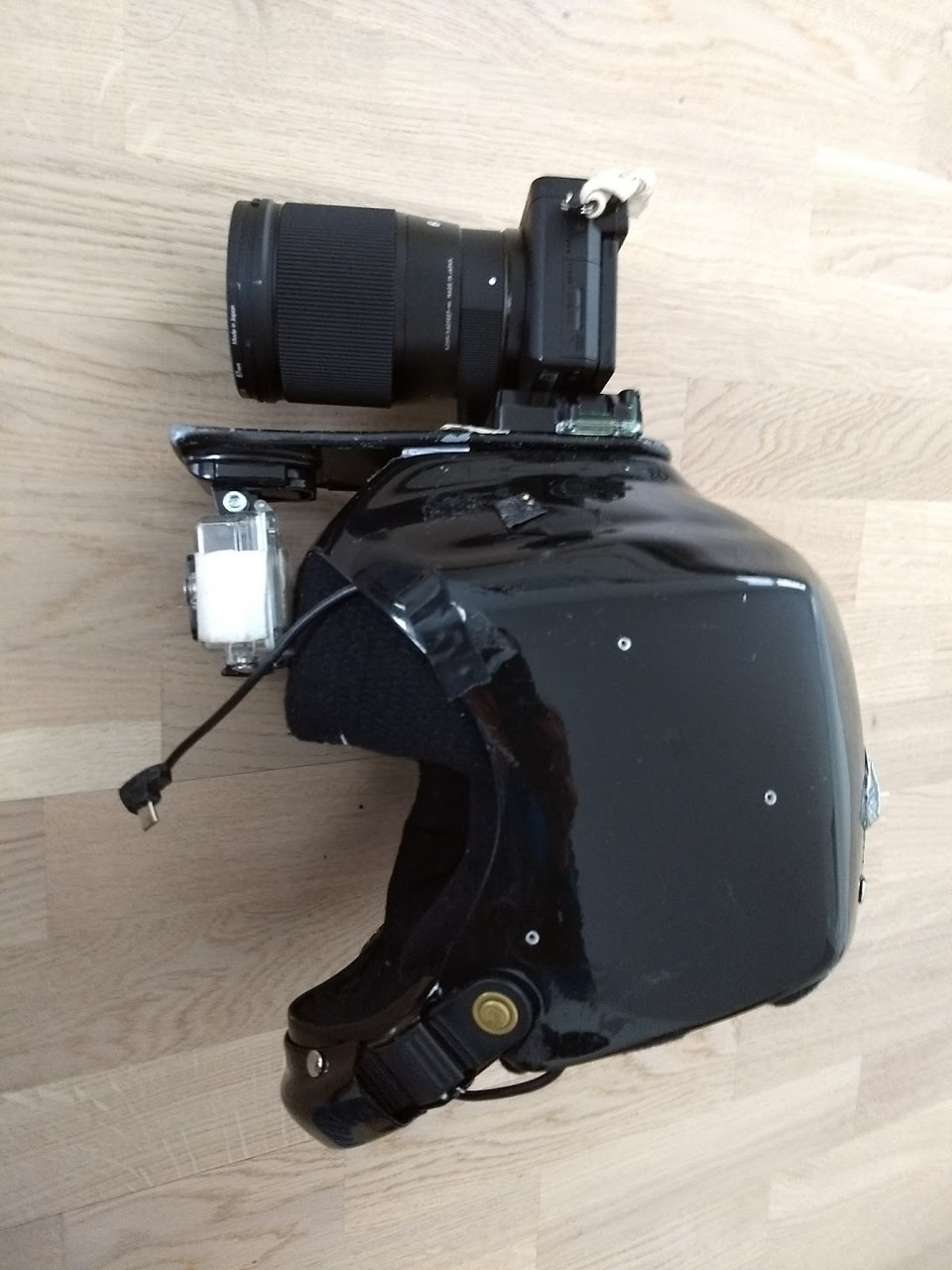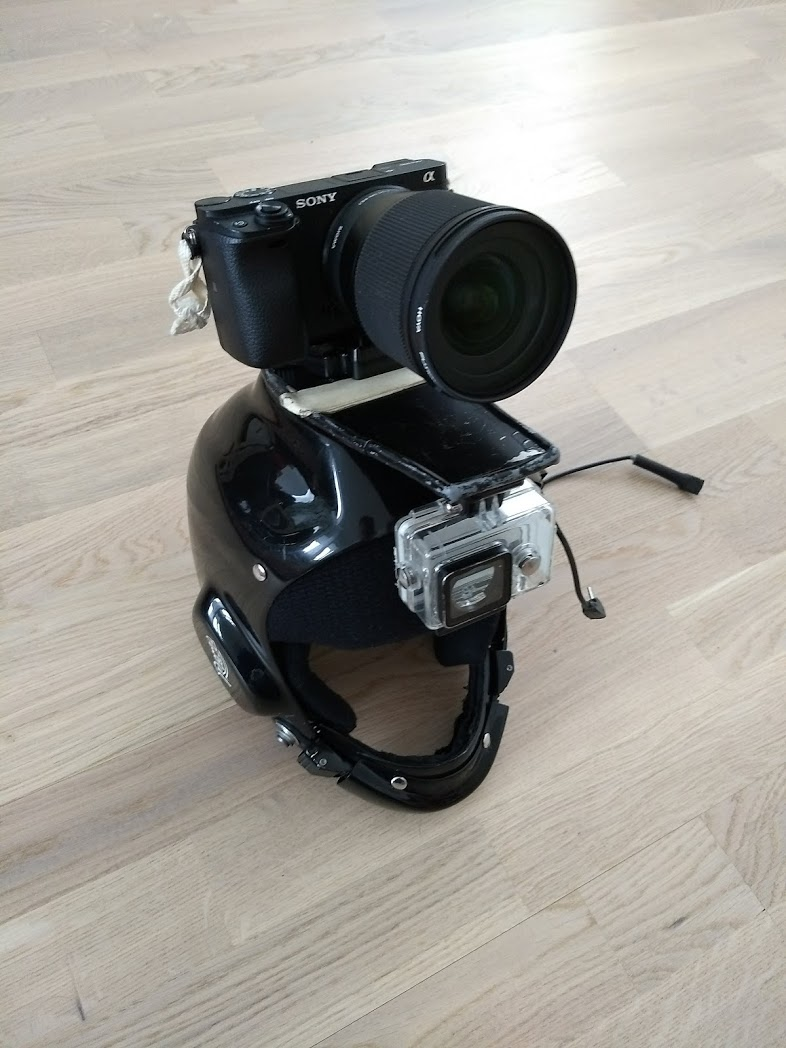But I did make a new model for Bell 2R mountain bike helmet which is completely snag proof. The new model is not adjustable and there is no easy way to get the camera out of the mount or to do a cut away, but the position and angle of the camera are very good for its purpose. I would recommend the mount+helmet combo for anyone wanting to have a very safe helmet. Unfortunately the mounting uses blu tack which makes it look very hackey. I would not recommend using the white one as it gets dirty in a week 🙂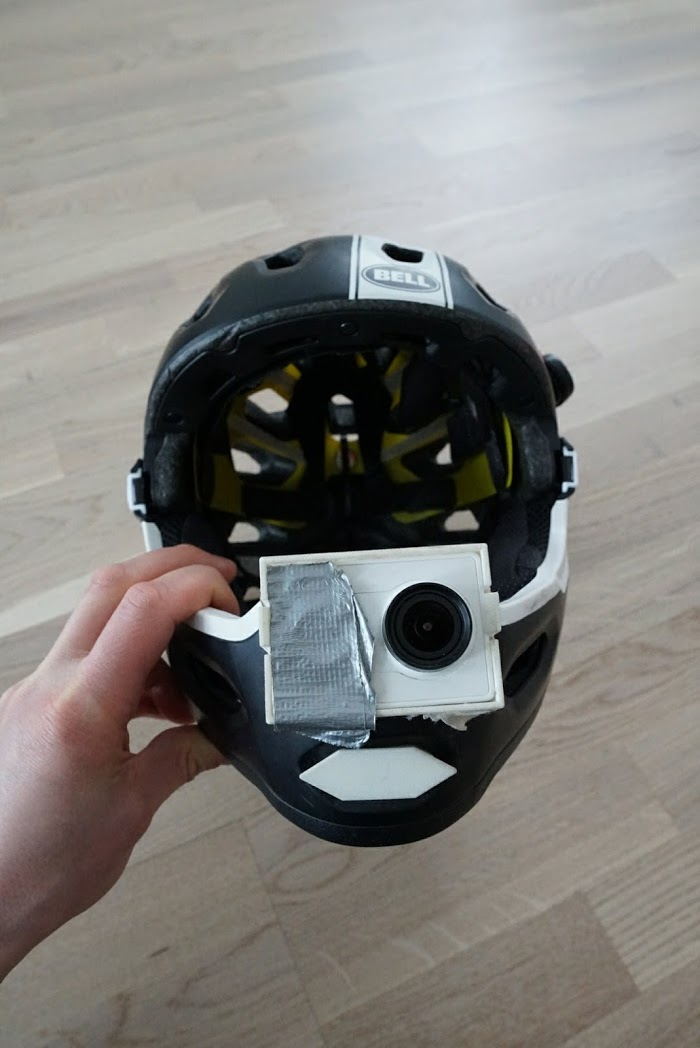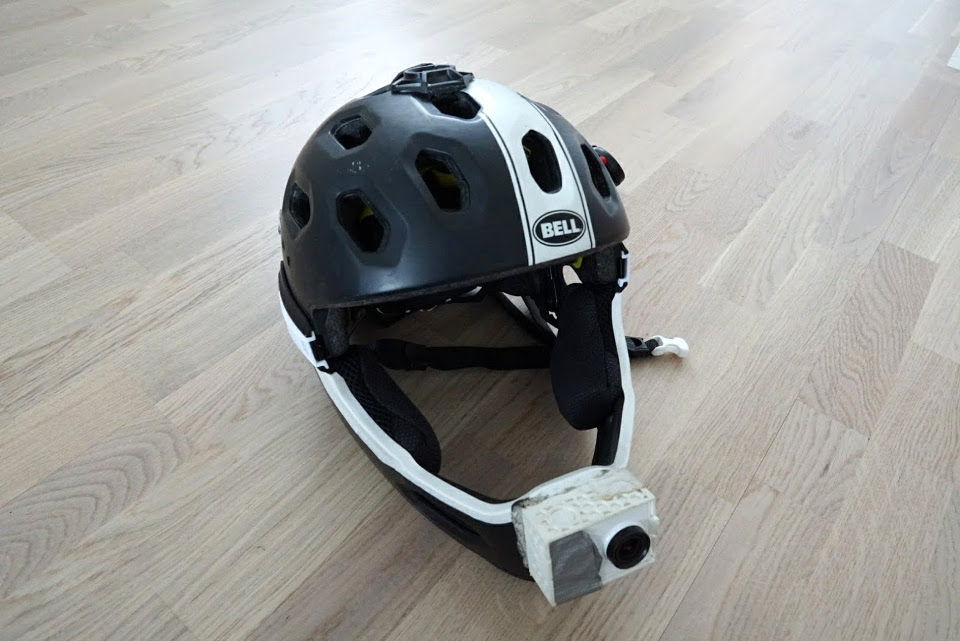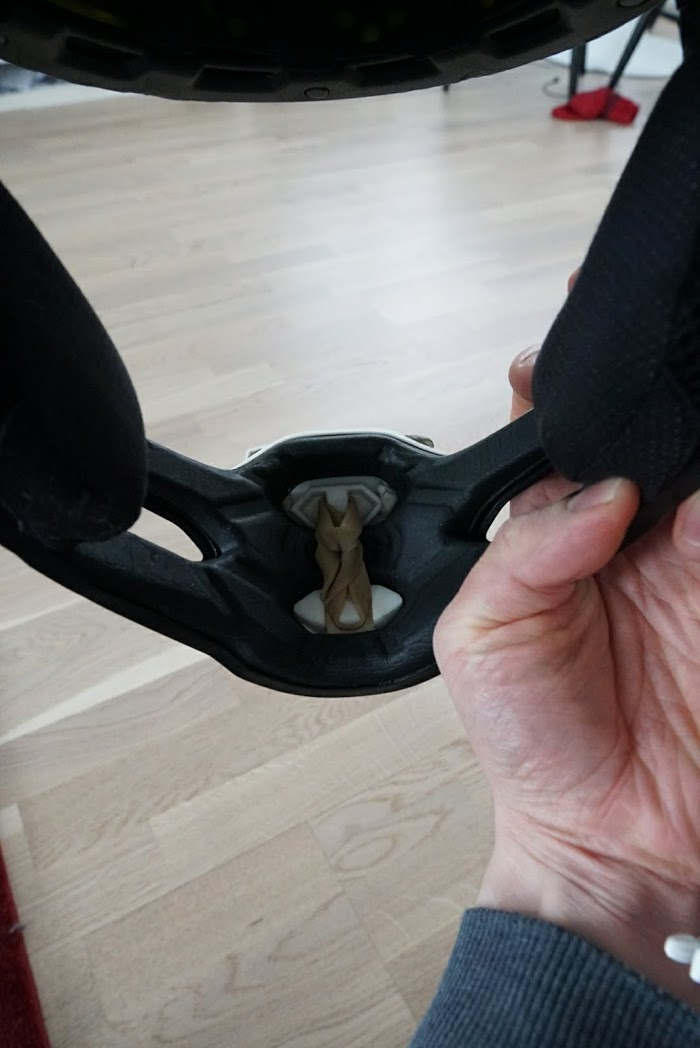There is also smallish flysight mount at the back of the helmet. The fit of the flysight mount is not very good, but its easy to use and serves its purpose.## Skydiving helmet chin mount

After getting skydiving C-license I have been allowed to carry camera during the jumps (see my jump videos at Youtube). Usually small action cameras are mounted on top of the helmet but as planes have quite restricted head room the camera is constantly exposed to small strikes. Also one of the most popular skydiving helmets, Cookie G3, does not have cutaway system which would allow the helmet to be quickly removed if the parachute is tangled to the camera.

I was looking for a camera for next season and I had hoped that new GoPro would have been announced before the new year but it seems that it will be delayed past start of the season. For mounting the camera I had decided that mounts made by Grellfab would be the safest option as the camera is mounted infront of the chin and the actual camera mount has cutaway system. Unfortunately as the Gopro was not announced and all Grellfab mounts have little bit different connection to the actual camera, I could not make the order.

Frustrated I decided to order cheap Chinese action camera Xiaomi Yi (~70€ instead of ~450€ for top of the line Gopro) and to 3D print the mounting system.

During the Christmas holidays I was able to reverse engineer the Cookie G3 chin piece and design mounting system for the Xiaomi Yi. I have not had change to study Grellfabs system so I had to make few wild guesses what would make functional design.

The final mounting system has three pieces, first one is plate which attaches to the helmet using rubber bands and cutaway pin. Third is the actual camera case/housing. Main reason for three piece system (pin+plate+case) instead of two piece (pin+mount) was that I had no idea for best camera angle. With two piece design the mount would protrude much less than with the adjustable one.

Modeling was done in Solidworks and the 3D prints were ordered from Sculpteo. I made the order on 2015-12-28 and the packet arrived 2016-01-05, really quick service! I’m quite happy with the quality, only quite annoying aspect is that all surfaces have expanded 0.05mm (things which should be 3.00mm are now 3.10mm). This casuses the rotating mechanism to be too tight and I had to use sandpaper to fit the parts together. On the other hand this was expected and as now the expansion is known it can be taken into account in future models.

The models have few mistakes which makes them rather annoying to use. The skeleton case with hexagon pattern is very firm over the camera. Unofrtunately I forgot to add way to push the camera out of the case. Now you need to pull all three restraining pins and then push the camera with a screwdriver. I think that one or two restraining pins would be enough.

Second mistake was made with the cutaway pin. Screw keeping the o-ring in place should be flush with the surface but the cut for the screw head was accidentally made 1mm too small. Another 3mm hole in the pin is intended for testing the structural strength of the pin.

The rotating mechanism uses four extrusion compared to Gopros two. Originally I thought that the material (“Strong and flexible” or Nylon) would be way too flexible and would need more support but after some comparisons to Gopros mechanism I think that two extrusions might be enough (although the Gopros material is little bit stiffer).

I hope that I will have time to make necessary changes and order new batch before start of the season. I also think that I will try to make few chin mounts for friends who have helmets for which Grellfab does not sell mounting. Also if I figure how to ship the mounts cheaply I might start selling them.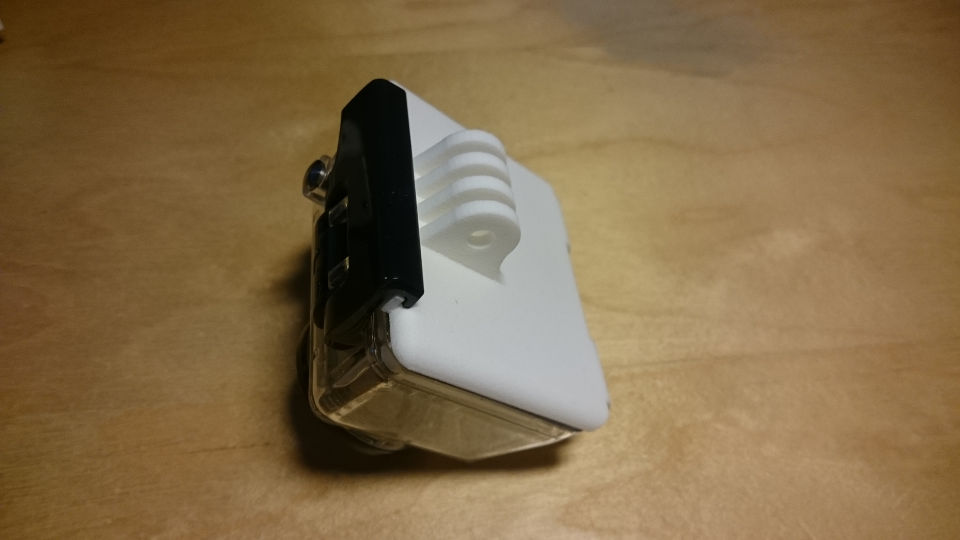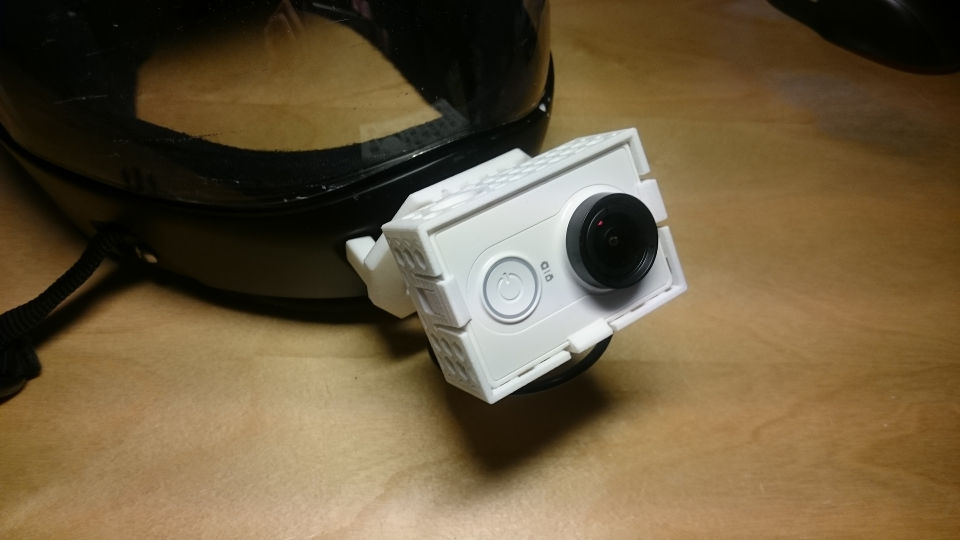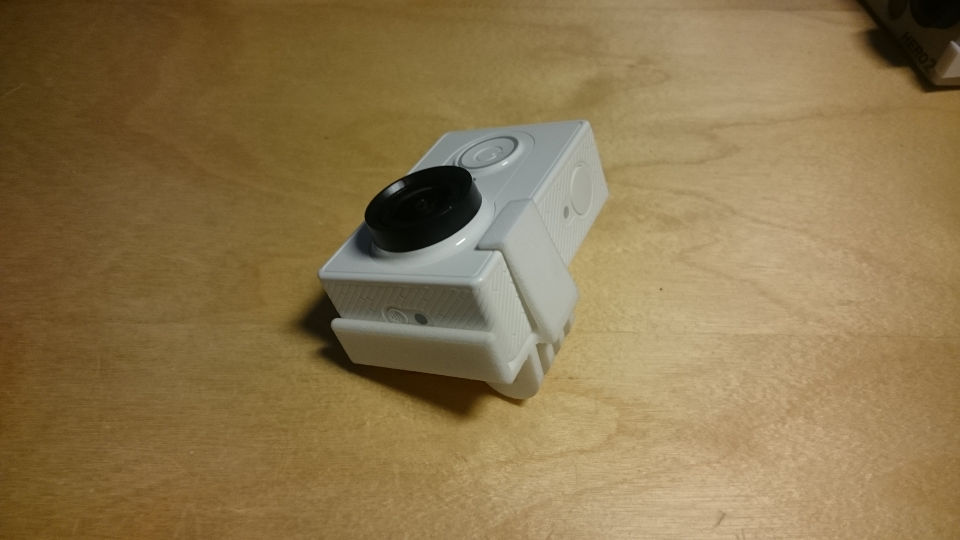## How image is formed

There exists different mathematical models for image formation, but for generic 3D measurement the most used one is the pinhole camera model. Pinhole camera model is often applied in machine vision applications because it is simple and accurate for camera systems with narrow angle of view. In the simplest pinhole camera model 3D points are directly projected to the plane of camera sensor after which optical distortion effect is applied to them. Even high quality optics has a non-ideal optical transfer function (OTF) which causes a single point to spread to a wider area on the camera sensor.

## Pinhole camera model

In pinhole camera model 3D points $\textbf{X}$ are projected trough single point in space (camera center $\mathbf{C}$ / focal point) to the camera sensor.

## Collection of links to free FPGA learning material

Note that I’m currently biased towards VHDL -> Xilinx -> Vivado.

## Camera position estimation from known 3D points

This article describes how to find camera matrix, including calibration matrix, from 6 or more known 3D points which have been projected to the camera sensor. Good reference for the article is in 1.

## Problem description

We know at least six 3D points in the scene ($X, Y$ and $Z$ coordinates) and their location at the camera sensor in pixel coordinates. We would like to find the location and orientation of the camera.

## Basics

If your object has 6 known points (known 3D coordinates, $X, Y$ and $Z$) you can compute the location of the camera related to the objects coordinate system.

First some basics.

Homogenous coordinate is vector presentation of euclidean coordinate $(X,Y,Z)$ in which we have appended so called scale factor $\omega$ such that the homogenous coordinate is $\textbf{X}=\omega \begin{bmatrix}X & Y & Z & 1\end{bmatrix}^T$. In your own calculations try to keep $\omega=1$ as often as possible (meaning that you “normalize” the homogenous coordinate by dividing it with its last element: $\textbf{X} \leftarrow \frac{\textbf{X}}{\omega}$). We can also use homogenous presentation for 2D points such that $\textbf{x}=\omega\begin{bmatrix}X & Y & 1\end{bmatrix}$ (remeber that these $\omega, X,Y$ and $Z$ are different for each point, be it 2D or 3D point). Homogenous coordinate presentation makes the math easier.

Camera matrix is $3\times4$ projection matrix from the 3D world to the image sensor:

$$\textbf{x}=P\textbf{X}$$

Where $\textbf{x}$ is the point on image sensor (with pixels units) and $\textbf{X}$ is the projected 3D point (lets say that it has millimeters as its units).

We remember that cross product between two 3-vectors can be defined as matrix-vector-multiplication such that:

$$\textbf{v} \times \textbf{u}=\\ ( \textbf{v} )_x \textbf{u}=\\ \begin{bmatrix} 0 & -v_3& v_2 \\ v_3 & 0 & -v_1 \\ -v_2 & v_1 & 0 \end{bmatrix} \textbf{u}$$

It is also useful to note that cross production $\textbf{v} \times \textbf{v}=\textbf{0}$.

Now lets try to solve the projection matrix $P$ from the previous equations. Lets multiply the projection equation from the left side with $\textbf{x}$s cross product matrix:

$$(\textbf{x})_x\textbf{x}=(\textbf{x})_xP\textbf{X}=\textbf{0}$$

Aha! The result must be zero vector. If we now open the equation we get:

$$\begin{bmatrix} 0 & -w& y \\ w & 0 & -x \\ -y & x & 0 \end{bmatrix} \begin{bmatrix} P_{1,1} & P_{1,2} & P_{1,3} & P_{1,4} \\ P_{2,1} & P_{2,2} & P_{2,3} & P_{2,4} \\ P_{3,1} & P_{3,2} & P_{3,3} & P_{3,4} \end{bmatrix} \textbf{X} \\=\begin{bmatrix} P_{3,4} W y – P_{2,1} X w – P_{2,2} Y w – P_{2,4} W w + P_{3,1} X y – P_{2,3} Z w + P_{3,2} Y y + P_{3,3} Z y \\ P_{1,4} W w + P_{1,1} X w – P_{3,4} W x + P_{1,2} Y w – P_{3,1} X x + P_{1,3} Z w – P_{3,2} Y x – P_{3,3} Z x \\ P_{2,4} W x + P_{2,1} X x – P_{1,4} W y – P_{1,1} X y + P_{2,2} Y x – P_{1,2} Y y + P_{2,3} Z x – P_{1,3} Z y \end{bmatrix}=\textbf{0}$$

With little bit of refactoring we can get the projection matrix $P$ outside of the matrix:

$$\tiny \begin{bmatrix} 0 & 0 & 0 & 0 & – X\, w & – Y\, w & – Z\, w & – W\, w & X\, y & Y\, y & Z\, y & W\, y\\ X\, w & Y\, w & Z\, w & W\, w & 0 & 0 & 0 & 0 & – X\, x & – Y\, x & – Z\, x & – W\, x\\ – X\, y & – Y\, y & – Z\, y & – W\, y & X\, x & Y\, x & Z\, x & W\, x & 0 & 0 & 0 & 0 \end{bmatrix} \begin{bmatrix} \textbf{P}_1 \\ \textbf{P}_2 \\ \textbf{P}_3 \\ \end{bmatrix}=\textbf{0}$$

Where $\textbf{P}_n$ is the transpose of $n$:th row of the camera matrix $P$. Last row of the previous (big) matrix equation is linear combination of the first two rows, so it does not bring any additional information and it can be left out.

Small pause so we can gather our toughs. Note that the previous matrix equation has to be formed for each known 3D->2D correspondence (there must be at least 6 of them).

Now, for each point correspondence, calculate the first two rows of the matrix above, stack the $2\times12$ matrices on top of each other and you get new matrix $A$ for which

$$A\begin{bmatrix} \textbf{P}_1 \\ \textbf{P}_2 \\ \textbf{P}_3 \\ \end{bmatrix}=\textbf{0}$$

As we have 12 unknowns and (at least) 12 equations this can be solved. Only problem is that we don’t want to have the trivial answer where
$$\begin{bmatrix} \textbf{P}_1 \\ \textbf{P}_2 \\ \textbf{P}_3 \\ \end{bmatrix}=\textbf{0}$$

Fortunately we can use singular value decomposition (SVD) to force

$$| \begin{bmatrix} \textbf{P}_1 \\ \textbf{P}_2 \\ \textbf{P}_3 \\ \end{bmatrix} |=1$$

So to solve the the equations, calculate SVD of matrix $A$ and pick the singular vector corresponding to the smallest eigen value. This vector is the null vector of matrix A and also the solution for the camera matrix $P$. Just unstack the $\begin{bmatrix} \textbf{P}_1 & \textbf{P}_2 & \textbf{P}_3 \end{bmatrix}^T$ and form $P$.

Now you wanted to know the distance to the object. $P$ is defined as:

$$P=K\begin{bmatrix}R & -R\textbf{C}\end{bmatrix}$$

where $\textbf{C}$ is the camera location relative to the objects origin. It can be solved from the $P$ by calculating $P$s null vector.

Finally, when you calculate the cameras location for two frames, you can calculate the unknown objects locations (or locations of some of the points of the object) by solving two equations for $X$:

$$\textbf{x}_1=P_1 \textbf{X} \\ \textbf{x}_2=P_2 \textbf{X} \\$$

Which goes pretty much the same way as how we solved the camera matrices:
$$(\textbf{x}_1)_xP_1\textbf{X}=\textbf{0} \\ (\textbf{x}_2)_xP_2\textbf{X}=\textbf{0} \\$$

And so on.

1. Harley, Zisserman – Multiple View Geometry 2004. Algorithm 7.1

## Camera geometry basics

This article tries to list minimum amount of math which is required for some of the other articles.

## Symbols used by this site

• Scalars are in italics
• $x$ and $y_0$ are scalars
• Vectors are bolded
• $\mathbf{x}$ and $\mathbf{\hat{x}}$ are vectors
• Matrices are usually upper case letters without italics or bolding
• $\mathrm{P}$ and $\mathrm{H}$ are matrices

Common symbols

• $\mathrm{P}$ is $3\times4$ projection matrix
• $\mathrm{P}=\mathrm{K}[\mathrm{R -R}\mathbf{C}]$
• $\mathrm{K}$ is $3\times3$ camera calibration matrix
• $\mathrm{R}$ is $3\times3$ rotation matrix
• $\mathbf{C}$ is $3\times1$ vector presenting camera center
• $\mathbf{T}$ is $3\times1$ vector presenting camera translation
• $\mathbf{T}=-\mathrm{R}\mathbf{C}$
• $\textbf{x}$ is $3\times1$ homogenous 2D point
• $\textbf{l}$ is $3\times1$ homogenous 2D line
• $\textbf{X}$ is $4\times1$ homogenous 3D point
• $\mathrm{H}$ is $3\times3$ homography/transformation matrix
• $\omega$ is scalar presenting the “scale” of homogenous coordinate

## Vector dot product

Vector dot product is defined for all vectors regardless of the dimension. For two same size vectors, $\mathbf{a}=[a_1, a_2, …, a_n]$ and $\mathbf{b}=[b_1, b_2, …, b_n]$, dot product is defined as $\mathbf{a} \cdot \mathbf{b}=\sum_{i=1}^n a_ib_i$.

Dot product has also geometric interpretation: if the two vectors are interpreted to exist in euclidean space the dot product then relates to cosine of the angle between the two vectors. More specifically $\mathbf{a} \cdot \mathbf{b}=|\mathbf A|\,|\mathbf B|\cos\theta$ where $\theta$ is the angle between the vectors.

This geometric interpretation can be useful for example when similarity between two vectors is compared, if the two vectors point to same direction, angle between the vectors is small and therefore $\cos\theta$ is large. If the angle between the vectors is large $\cos\theta$ is small (or zero if the vectors are prependicular).

(1)

## Vector cross product

Vector cross product is defined for two 3-vectors and it produces new vector which is perpendicular to both of the vectors. As now the angle of the new vector is 90° to both original vectors, this means that dot product between the new and old vectors is zero.

Cross product between two 3-vectors, $\mathbf{v}=[v_1, v_2, v_3]$ and $\mathbf{u}=[u_1, u_2, u_3]$, is defined as:
$$\mathbf{u} \times \mathbf{v}=\begin{bmatrix} u_2v_3 – u_3v_2 \\ u_3v_1 – u_1v_3 \\ u_1v_2 – u_2v_1 \\ \end{bmatrix}^T$$
Note that this can also be presented in matrix form:
$$\mathbf{v} \times \mathbf{u}=[ \mathbf{v} ]_\times \mathbf{u}=\begin{bmatrix} 0 & -v_3& v_2 \\ v_3 & 0 & -v_1 \\ -v_2 & v_1 & 0 \end{bmatrix} \mathbf{u}$$

(2, 3)

## Homogenous coordinates

Homogenous coordinates are system of coordinates which is commonly used for projective geometry instead of Cartasian coordinates. Instead of using two scalars to present 2D point in homogenous coordinates 2D point is presented using 3 scalars. For example 2D point $\mathbf{x}$ which is in Cartesian coordinates presented as $(x,y)$ is presented in homogenous
coordinates as:
$$\mathbf{x}=\begin{bmatrix} \omega x \\ \omega y \\ \omega \end{bmatrix}=\omega \begin{bmatrix} x \\ y \\ 1 \end{bmatrix}$$

Homogenous coordinates are used to present both 2D and 3D points. 3D points are just 4-vectors: $\mathbf{X}=\omega [x,y,z,1]^T$

### Why should we use homogenous coordinates?

Homogenous coordinates make it easier to handle projective geometry.

For example lets try to translate non-homogenous 2D point $\mathbf{x_{2d}}=[x,y]^T$ two units to the positive x-direction using matrix multiplication with matrix
$$\mathrm{H_{2d}}=\begin{bmatrix} h_{1,1} & h_{1,2} \\ h_{2,1} & h_{2,2} \end{bmatrix}$$
$$\mathbf{x_{translated}}=\mathrm{H_{2d}}\mathbf{x_{2d}}=\begin{bmatrix} h_{1,1} & h_{1,2} \\ h_{2,1} & h_{2,2} \end{bmatrix} \begin{bmatrix} x \\ y \end{bmatrix}=\begin{bmatrix} h_{1,1}x+h_{1,2}y \\ h_{2,1}x+h_{2,2}y \\ \end{bmatrix}$$
As all elements of $\mathbf{x_{translated}}$ depend on $\mathrm{H_{2d}}$ it is easy to see that 2D translation can not be done with $2\times2$ matrix multiplication.

But if we use homogenous coordinates and $3\times3$ transformation matrix we can define translation as:
$$\mathrm{H}\mathbf{x}=\begin{bmatrix} 1 & 0 & \delta x \\ 0 & 1 & \delta y \\ 0 & 0 & 1 \\ \end{bmatrix} \omega \begin{bmatrix} x \\ y \\ 1 \\ \end{bmatrix}=\omega \begin{bmatrix} x + \delta x \\ y + \delta y \\ 1 \\ \end{bmatrix}$$

If after transformation we get homogenous coordinate for which the $\omega$ is not $1$, we can simply divide the result with $\omega$ and get more easily read presentation of the point/line.

(4, 5)

## 2D Line

Useful way to define a line is: $\mathbf{l}=[a, b, c]^T$, point $\mathbf{x}$ is on the line if $\mathbf{x} \cdot \mathbf{l}=0$ or $ax + by + c=0$.

If we need to find line $\mathbf{l}$ which travels trough two 2D points, $\mathbf{x_1}$ and $\mathbf{x_2}$, it can be found easily by taking cross product of the two points: $\mathbf{l}=\mathbf{x_1} \times \mathbf{x_2}$. Result can be easily verified if we rember the properties of the cross product and dot product:

• Cross product results in a vector which is perpendicular to both original vectors.
• Dot product results is scalar which is directly proportional to the cosine of the angle between the two vectors.
• If the two orignal vectors are perpendicular the result is 0.

Same way it easy to find point $\mathbf{x}$ in which two lines, $\mathbf{l_1}$ and $\mathbf{l_2}$ cross each other: $\mathbf{x}=\mathbf{l_1} \times \mathbf{l_2}$.

(4)